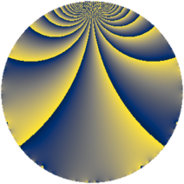# Properties

 Label 855.2.cLevel $855$ Weight $2$ Character orbit 855.c Rep. character $\chi_{855}(514,\cdot)$ Character field $\Q$ Dimension $44$ Newform subspaces $7$ Sturm bound $240$ Trace bound $5$

# Related objects

## Defining parameters

 Level: $$N$$ $$=$$ $$855 = 3^{2} \cdot 5 \cdot 19$$ Weight: $$k$$ $$=$$ $$2$$ Character orbit: $$[\chi]$$ $$=$$ 855.c (of order $$2$$ and degree $$1$$) Character conductor: $$\operatorname{cond}(\chi)$$ $$=$$ $$5$$ Character field: $$\Q$$ Newform subspaces: $$7$$ Sturm bound: $$240$$ Trace bound: $$5$$ Distinguishing $$T_p$$: $$2$$, $$11$$

## Dimensions

The following table gives the dimensions of various subspaces of $$M_{2}(855, [\chi])$$.

Total New Old
Modular forms 128 44 84
Cusp forms 112 44 68
Eisenstein series 16 0 16

## Trace form

 $$44 q - 42 q^{4} + q^{5} + O(q^{10})$$ $$44 q - 42 q^{4} + q^{5} - 12 q^{10} + 2 q^{11} - 4 q^{14} + 62 q^{16} + 4 q^{19} - 8 q^{20} + 25 q^{25} + 20 q^{26} + 8 q^{29} - 8 q^{31} - 40 q^{34} + q^{35} + 16 q^{40} - 16 q^{41} - 20 q^{44} + 68 q^{46} - 66 q^{49} + 16 q^{50} + 11 q^{55} - 28 q^{56} - 28 q^{59} - 2 q^{61} - 102 q^{64} + 16 q^{65} - 24 q^{70} - 12 q^{71} + 36 q^{74} - 10 q^{76} + 26 q^{80} + 9 q^{85} + 52 q^{86} + 36 q^{89} + 64 q^{91} + 20 q^{94} + q^{95} + O(q^{100})$$

## Decomposition of $$S_{2}^{\mathrm{new}}(855, [\chi])$$ into newform subspaces

Label Dim $A$ Field CM Traces $q$-expansion
$a_{2}$ $a_{3}$ $a_{5}$ $a_{7}$
855.2.c.a $2$ $6.827$ $$\Q(\sqrt{-1})$$ None $$0$$ $$0$$ $$-4$$ $$0$$ $$q+iq^{2}+q^{4}+(-2-i)q^{5}+2iq^{7}+\cdots$$
855.2.c.b $2$ $6.827$ $$\Q(\sqrt{-1})$$ None $$0$$ $$0$$ $$2$$ $$0$$ $$q+iq^{2}+q^{4}+(1+2i)q^{5}+2iq^{7}+\cdots$$
855.2.c.c $2$ $6.827$ $$\Q(\sqrt{-1})$$ None $$0$$ $$0$$ $$4$$ $$0$$ $$q+iq^{2}+q^{4}+(2-i)q^{5}-2iq^{7}+3iq^{8}+\cdots$$
855.2.c.d $6$ $6.827$ 6.0.16516096.1 None $$0$$ $$0$$ $$1$$ $$0$$ $$q-\beta _{5}q^{2}+(-1+\beta _{2})q^{4}+(-\beta _{3}+\beta _{5})q^{5}+\cdots$$
855.2.c.e $6$ $6.827$ 6.0.350464.1 None $$0$$ $$0$$ $$0$$ $$0$$ $$q-\beta _{4}q^{2}+(-\beta _{1}+\beta _{2})q^{4}+(-\beta _{1}-\beta _{4}+\cdots)q^{5}+\cdots$$
855.2.c.f $12$ $6.827$ $$\mathbb{Q}[x]/(x^{12} - \cdots)$$ None $$0$$ $$0$$ $$0$$ $$0$$ $$q+\beta _{9}q^{2}+(-1+\beta _{2})q^{4}-\beta _{8}q^{5}-\beta _{6}q^{7}+\cdots$$
855.2.c.g $14$ $6.827$ $$\mathbb{Q}[x]/(x^{14} + \cdots)$$ None $$0$$ $$0$$ $$-2$$ $$0$$ $$q+\beta _{1}q^{2}+(-2+\beta _{2})q^{4}+\beta _{10}q^{5}+\cdots$$

## Decomposition of $$S_{2}^{\mathrm{old}}(855, [\chi])$$ into lower level spaces

$$S_{2}^{\mathrm{old}}(855, [\chi]) \cong$$ $$S_{2}^{\mathrm{new}}(45, [\chi])$$$$^{\oplus 2}$$$$\oplus$$$$S_{2}^{\mathrm{new}}(95, [\chi])$$$$^{\oplus 3}$$$$\oplus$$$$S_{2}^{\mathrm{new}}(285, [\chi])$$$$^{\oplus 2}$$